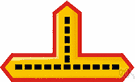# intersection point

Also found in: Thesaurus, Acronyms, Encyclopedia, Wikipedia.
ThesaurusAntonymsRelated WordsSynonymsLegend:
 Noun 1intersection point - a point where lines intersect  point - a geometric element that has position but no extension; "a point is defined by its coordinates"metacenter, metacentre - (shipbuilding) the point of intersection between two vertical lines, one line through the center of buoyancy of the hull of a ship in equilibrium and the other line through the center of buoyancy of the hull when the ship is inclined to one side; the distance of this intersection above the center of gravity is an indication of the stability of the shipvertex - the point of intersection of lines or the point opposite the base of a figure
Based on WordNet 3.0, Farlex clipart collection. © 2003-2012 Princeton University, Farlex Inc.
Translations
References in periodicals archive ?
As it was described in previous section, it is necessary to perform a multi-stage adjustment, which will ensure the rotation of the reflector around the intersection point of the module axes.
The intersection points between traffic line and top and bottom horizontal lines ([p.sup.t] and [p.sup.b]) can be calculated using the equation of straight line set up by [p.sub.1] and [p.sub.2].
Then g is a continuous map, and we obtain a continuous involution f: L [right arrow] L by sending y [member of] L to the second intersection point of g(y) and L, or to y itself if g(y) happens to touch L.
Depending on the position of the object with respect to the ray shooting point, the exact face is chosen for finding the intersection point. As an example, if an object is in the first quadrant then the ray will possibly hit the left or back surface of that object and in that case, only these surfaces will be used for finding the intersection point, as demonstrated in Figure 6(b).
An intersection point is calculated using the topological relation between two intersection planes.
Let G = ([x.sub.g], [t.sub.g]) be the intersection point of the lines
Proposition (Pappus): If the three sides of a variable triangle are passing through three fixed, collinear points and two of its vertices are moving along two fixed lines, then the third vertex is moving along a line, which is passing through the intersection point of the first two lines.
HE Essa Kazim, Governor of DIFC and Chairman of the DIFC Authority Board of Directors, said that the DIFC is at an intersection point in Dubai's history, not only as a global economic centre, but also given the strong foundations it has built for a sustainable economy.
* The intersection point that is covered by more than one nodes is not a hole boundary point; otherwise it is a hole boundary point.
"5G will be the intersection point of IoT and mobility and its deployments will multiply the growth of IoT," he said.
The location of point A as shown in Figure 2 is corresponding to the intersection point of a plan view of the existing tunnel and northbound tunnel of Line 4.
The calibration is to determine the intrinsic and extrinsic parameters of the camera and the projector, and the reconstruction is usually based on the ray triangulation principle by computing the intersection point.

Site: Follow: Share:
Open / Close